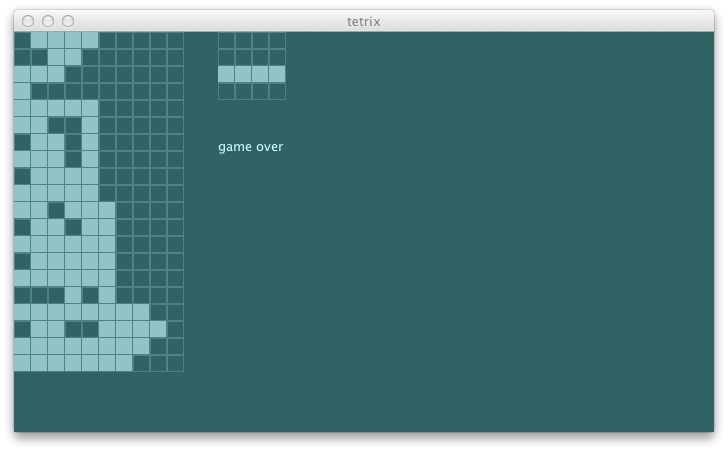### heuristic function

Plan B is to introduce a heuristic function.

h(n) = estimated cost of cheapest path from node n to a goal node.

Technically speaking we don’t have a goal node, so the term may not apply here, but the idea is to approximate the situation to nudge tree searching to a right direction. In our case, we can think of it as having some penalty for bad shapes. For example, let’s add penalty for having more than one height difference between the columns. We should square the gaps to make the penalty harsher.

``````                                                                              s2"""
penalize having gaps between the columns                                  \$utility4
"""
...
def utility4 = {
val s = newState(Seq(
(0, 0), (0, 1), (0, 2), (0, 3), (0, 4), (0, 5), (0, 6))
map { Block(_, TKind) }, (10, 20), TKind :: TKind :: Nil)
agent.utility(s) must_== -36.0
}
``````

The test fails as expected:

``````[info] Utility function should
[info] + evaluate initial state as 0.0,
[info] + evaluate GameOver as -1000.0,
[info] + evaluate an active state by lineCount
[error] x penalize having gaps between the columns
[error]    '0.0' is not equal to '-36.0' (AgentSpec.scala:10)
``````

Let’s use the REPL to figure this one out. Type `console` from sbt.

``````Welcome to Scala version 2.10.2 (Java HotSpot(TM) 64-Bit Server VM, Java 1.6.0_51).
Type in expressions to have them evaluated.

scala> import com.eed3si9n.tetrix._
import com.eed3si9n.tetrix._

scala> import Stage._
import Stage._

scala> val s = newState(Seq(
(0, 0), (0, 1), (0, 2), (0, 3), (0, 4), (0, 5), (0, 6))
map { Block(_, TKind) }, (10, 20), TKind :: TKind :: Nil)
s: com.eed3si9n.tetrix.GameState = GameState(List(Block((0,0),TKind), Block((0,1),TKind),
Block((0,2),TKind), Block((0,3),TKind), Block((0,4),TKind), Block((0,5),TKind),
Block((0,6),TKind), Block((4,18),TKind), Block((5,18),TKind), Block((6,18),TKind),
Block((5,19),TKind)),(10,20),
Piece((5.0,18.0),TKind,List((-1.0,0.0), (0.0,0.0), (1.0,0.0), (0.0,1.0))),
Piece((2.0,1.0),TKind,List((-1.0,0.0), (0.0,0.0), (1.0,0.0), (0.0,1.0))),
List(),ActiveStatus,0)

scala> s.blocks map {_.pos} groupBy {_._1}
res0: scala.collection.immutable.Map[Int,Seq[(Int, Int)]] = Map(
5 -> List((5,18), (5,19)), 4 -> List((4,18)), 6 -> List((6,18)),
0 -> List((0,0), (0,1), (0,2), (0,3), (0,4), (0,5), (0,6)))
``````

This is not good. We have the current piece loaded in `s.blocks`. But the `unload` is currently a private method within `Stage` object. We can refactor it out to `GameState` class as follows:

``````case class GameState(blocks: Seq[Block], gridSize: (Int, Int),
currentPiece: Piece, nextPiece: Piece, kinds: Seq[PieceKind],
status: GameStatus, lineCount: Int) {
def view: GameView = ...
def unload(p: Piece): GameState = {
val currentPoss = p.current map {_.pos}
this.copy(blocks = blocks filterNot { currentPoss contains _.pos })
}
this.copy(blocks = blocks ++ p.current)
}
``````

With minor changes to `Stage` object, all tests run expect for the current one. Now REPL again:

``````... the same thing as above ...

res0: com.eed3si9n.tetrix.GameState = GameState(List(Block((0,0),TKind),
Block((0,1),TKind), Block((0,2),TKind), Block((0,3),TKind), Block((0,4),TKind),
Block((0,5),TKind), Block((0,6),TKind)),(10,20),
Piece((5.0,18.0),TKind,List((-1.0,0.0), (0.0,0.0), (1.0,0.0), (0.0,1.0))),
Piece((2.0,1.0),TKind,List((-1.0,0.0), (0.0,0.0), (1.0,0.0), (0.0,1.0))),List(),ActiveStatus,0)

scala> s.unload(s.currentPiece).blocks map {_.pos} groupBy {_._1}
res1: scala.collection.immutable.Map[Int,Seq[(Int, Int)]] = Map(
0 -> List((0,0), (0,1), (0,2), (0,3), (0,4), (0,5), (0,6)))

scala> val heights = s.unload(s.currentPiece).blocks map {_.pos} groupBy {_._1} map {
case (k, v) => (k, v.map({_._2}).max) }
heights: scala.collection.immutable.Map[Int,Int] = Map(0 -> 6)

scala> heights.getOrElse(1, 0)
res6: Int = 0

scala> (0 to s.gridSize._1 - 2)
res7: scala.collection.immutable.Range.Inclusive = Range(0, 1, 2, 3, 4, 5, 6, 7, 8)

scala> val gaps = (0 to s.gridSize._1 - 2).toSeq map { x =>
heights.getOrElse(x, 0) - heights.getOrElse(x + 1, 0) }
gaps: scala.collection.immutable.IndexedSeq[Int] = Vector(6, 0, 0, 0, 0, 0, 0, 0, 0)

scala> val gaps = (0 to s.gridSize._1 - 2).toSeq map { x =>
heights.getOrElse(x, 0) - heights.getOrElse(x + 1, 0) } filter {_ > 1}
gaps: scala.collection.immutable.IndexedSeq[Int] = Vector(6)

scala> gaps map {x => x * x} sum
res5: Int = 36
``````

I did a lot more typos and experiments than above. But you get the idea. We can incrementally construct expression using the REPL by chaining one operation after another. When we get the answer, copy-past it into the editor:

``````  def utility(state: GameState): Double =
if (state.status == GameOver) minUtility
else state.lineCount.toDouble - penalty(state)
private[this] def penalty(s: GameState): Double = {
val heights = s.unload(s.currentPiece).blocks map {_.pos} groupBy {
_._1} map { case (k, v) => (k, v.size) }
val gaps = (0 to s.gridSize._1 - 2).toSeq map { x =>
heights.getOrElse(x, 0) - heights.getOrElse(x + 1, 0) } filter {_ > 1}
gaps map {x => x * x} sum
}
``````

The tests pass.

``````[info]   Utility function should
[info]     + evaluate initial state as 0.0,
[info]     + evaluate GameOver as -1000.0,
[info]     + evaluate an active state by lineCount
[info]     + penalize having gaps between the columns
``````

There’s another problem with the current solver. Except for `Drop` the current piece is hovering midair, so it cannot be part of the evaluation. To solve this, we can simply append `Drop` unless it’s already dropped. I am going to change the implementation and see which test would fail:

``````  def bestMove(s0: GameState): StageMessage = {
var retval: StageMessage = MoveLeft
var current: Double = minUtility
possibleMoves foreach { move =>
val ms =
if (move == Drop) move :: Nil
else move :: Drop :: Nil
val u = utility(Function.chain(ms map {toTrans})(s0))
if (u > current) {
current = u
retval = move
} // if
}
retval
}
``````

Composing the transition function with `Function.chain` again. Now let’s run the test.

``````[info] Solver should
[info] + pick MoveLeft for s1
[error] x pick Drop for s3
[error]    'Tick' is not equal to 'Drop' (AgentSpec.scala:14)
``````

This is not surprising. Since we added `Drop` at the end, there’s no difference between `Tick` and `Drop` anymore. We can fix this by relaxing the spec:

``````  def solver2 =
agent.bestMove(s3) must beOneOf(Drop, Tick)
``````

Now the agent started to pick moves other than `MoveLeft`, but it’s preferring the left side of the grid a lot more.Deepening the search tree should hopefully make things better. We’ll get back to this tomorrow.

``````\$ git fetch origin
\$ git co day7v2 -b try/day7
\$ sbt swing/run
``````